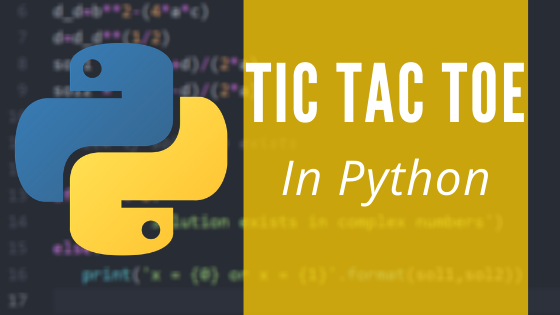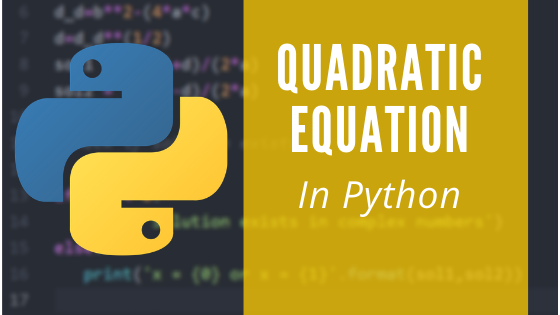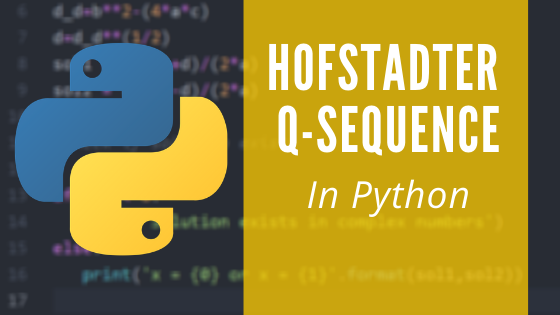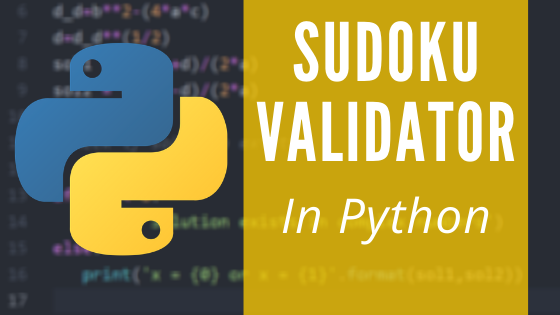## Tag Archive : Rock-Paper-Scissors game not working – Python

In this article, we have built a program that plays rock paper scissor, with the Python code. Please install python 3.8 from the link given below to run this code.

I prefer you to use atom text editor to type your code or to save the given code:
https://atom.io/

##### Recommended:The Classic Tic-Tac-Toe with Python

Link for the code in GitHub

## Example Input:

``R``

## Output:

``````You Win!!!
~~CONGRATS~~
You:R
Computer:S``````

## Example Input:

``R``

## Output:

``````===========
====TIE====
===========``````
##### Recommended:Quadratic Equation Code in Python

## Example Input:

``R``

## Output:

``````You Lose!!!
~~TRY AGAIN:-(~~
You:P
Computer:S``````

## Example Input:

``Something else``

## Output:

``````=====Error=====
Input Format: R
or
Input Format: S
or
Input Format: P``````

## Code:

``````x = input().upper()
y = {'R','P','S'}.pop()
if (x=='R'and y=='S') or (x=='S' and y=='P')or(x=='P'and y=='R'):
print(f"You Win!!!\n~~CONGRATS~~\n You:{x}\n Computer:{y}")
elif (y=='R'and x=='S') or (y=='S' and x=='P')or(y=='P'and x=='R'):
print(f"You Lose!!!\n~~TRY AGAIN:-(~~\n You:{x}\n Computer:{y}")
elif x==y:print('===========\n====TIE====\n===========')
else:print('=====Error=====\nInput Format: R\n\tor\nInput Format: S\n\tor\nInput Format: P')
``````
##### Recommended:Hofstadter Q-Sequence in Python

## Code Explanation:

In this code, we get input from the user, which is turned to upper case using the function upper() to avoid case confusion.

In order to store a random value for y, we use pop() function to a set.

As per the conditions of the game, we use if, elif and else statement that prints “You Win!!!…” if user wins and “You Lose!!!…” if not.

If equal values are received from both the sides, the code prints out “TIE”.

If the user enters anything other than R,P or S, the code prints out the input format.

And now, our code works perfectly fine.

##### Recommended:Sudoku Validator using Python

## Conclusion:

The above article explains the Rock Paper Scissor game using python coding. If you want to download the code from GitHub, click here. If you have any questions or feedback on this, feel free to post in the comments section below.